PLT Redex & SEwPR

Errata

Page 6, first sentence below first box: "We take it as a short-hand for following constraints" should be "We take it as a short-hand for the following constraints" Thanks to Paul Ojanen.

Page 18, in the first subcase of the first inductive case of the proof for Lemma 2.4, the if statements are reversed. This is the correct case: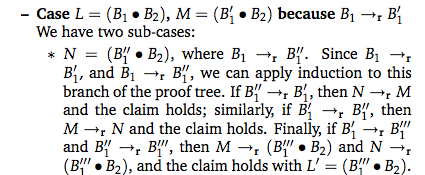Thanks to Stephen Chang.

Also on page 18, the first subcase of the first inductive case, the sentence "for some B'_0 and B_0 r B'_0" is wrong. It is deleted in the revised version. Thanks to Abel Nieto Rodriguez.

Pages 26 and 46, the substitution functions should have had the condition X3 != X2 in the second lambda case. These are the corrected figures: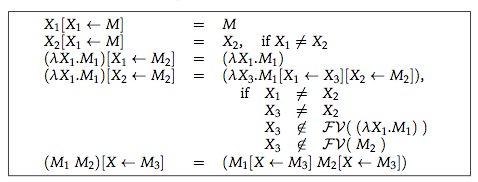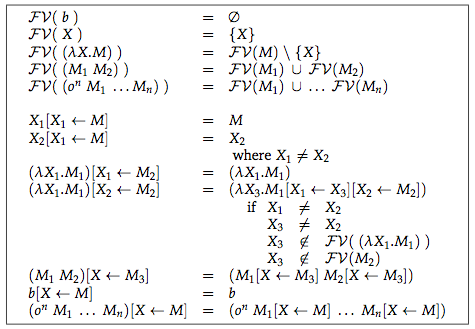Thanks to Saliya Ekanayake for pointing this out.

Page 40, Lemma 3.4 should have -->>n instead of -->>r in the statement of Church-Rosser.

Page 43, last sentence of section 3.10: "Rosser, another one Church's students" should be "Rosser, another one of Church's students" Thanks to Paul Ojanen.

Page 57, in the first case of the proof of Lemma 4.7, the second N should be N'. Thanks to Stephen Chang.

Page 58, In Exercise 4.5 X ∉ FV(L) should be X' ∉ FV(L). Thanks to Hannah Quay-de la Vallee.

Page 64, exercise 4.10, first sentence: "Design a variant of \iswim\ and that uses call-by-name to pass parameters." should be "Design a variant of \iswim\ that uses call-by-name to pass parameters." Thanks to Paul Ojanen.

Page 67, Lemma 5.1 is incorrectly referred to as "Theorem 5.1".

Page 69, "reductions steps" should be "reduction steps".

Page 72, the proof of Lemma 5.5 uses Lemma 5.6 incorrectly. The second (bullet) case should state that M |-->>_v K, not just in single-step mode. It follows that, if M needs m steps to standard reduce to K, the desired standard reduction is prefixed by the corresponding m terms, not just M. Thanks to Tony Garnock-Jones, the 2010 class of John Clements (CalPoly), and Vishesh Yadav.

Page 73, the statement of the first full case of Lemma 5.5 on this page should use N'i instead of Ni: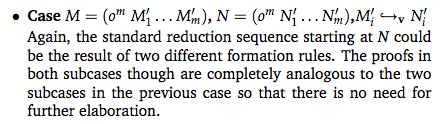Thanks to Stephen Chang.

Page 73, the statement of the third case of Lemma 5.6. should use J instead of L (to avoid confusion with the term L in the statement of the lemma). Furthermore, the result of applying the induction hypothesis yields a reduction sequence that ends in L (not N):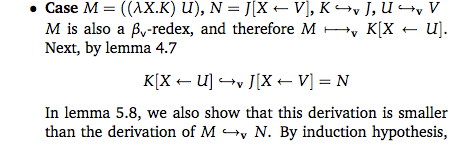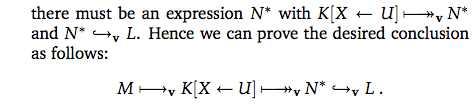Thanks to Stephen Chang.

Page 75, the calculation introduced with "Putting it all together, we get" should be as follows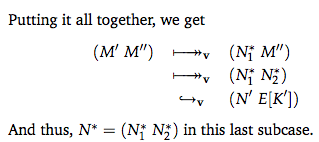Thanks to Stephen Chang.

Page 75, the statement of the first full case of Lemma 5.6 on this page should use N'i instead of Ni. Thanks to Stephen Chang.

Page 76, the undefined variables s denotes the size of the constructed term. Thanks to Stephen Chang.

Page 77, third subcase and the last case should use capitalized "Y" not lower-case "y." Thanks to Stephen Chang.

Page 79, the statement of the first full case of Lemma 5.8 on this page should use N'i instead of Ni. Thanks to Stephen Chang.

Page 79, the proof of Lemma 5.2 should refer to theorem 5.4, not 5.5. Thanks to Stephen Chang.

Page 82, Proof for Lemma 5.10 refers to "theorem" 5.1. This should be a reference "lemma" 5.1. Thanks to Stephen Chang.

Page 83, Proof for Lemma 5.10, case 2b uses ... [x <- ...] instead of ... [X <- ...] Thanks to Vishesh Yadav.

Page 84, Proof for Lemma 5.11 mentions the "assumption that C[Ω] is undefined." This should say "assumption that evalv(C[Ω]) is undefined." Thanks to Stephen Chang.

Page 86, line 2: "(case 3)" should be "(case 2)". Thanks to Stephen Chang.

Page 99, line 2, between the two boxes: "Hence the machine is called CK machine" should be "Hence the machine is called the CK machine". Thanks to Paul Ojanen.

Page 100, line 1: "SCC ad CK machine" should be "SCC and CK machines". Thanks to Paul Ojanen.

Pages 141 through 143, section 8.4 (part I) contains a major flaw. The notions of reductions on page 142 do not satisfy the Church-Rosser lemma and therefore theorem 8.17 is false as stated. Here is a counter-example:

catch (+ (throw 0) 1) with (λxy.x)
Using the reductions of page 142, it is possible to reduce this term to both 0 and 1 using the calculations
 catch (+ (throw 0) 1) with (λxy.yx) = catch (throw 0) with (λxy.yx) by throw = (λxy.yx) 0 (λy.y) by cntrl = (λy.y) 0 by βv = 0 by βv
and
 catch (+ (throw 0) 1) with (λxy.x) = (λxy.x) 0 (λy.(+ y 1)) by cntrl = (λy.(+ y 1)) 0 by βv = (+ 0 1) by βv = 1 by δ

We can fix this problem with the elimination of throw from the first box on page 142. This change induces changes to the definitions of the evaluation functions on page 142 and 143 as well as the definitions of the standard reduction function. To avoid any confusion, here is the revised pages 141 through 143.

Thanks to Ryan Culpepper for discovering this major problem.

Page 165, the left-hand side of the [csfis] rule should read:

`<E[((λX.M) V)],Σ>`
Thanks to Prabhakar Ragde for reporting this error.

Page 170, the side-condition on the [csgc] rule is incorrect. It should be this: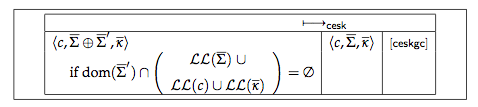Thanks to David Van Horn.

Page 172, "<M,Ebar>" on the right hand side of the [ceksgc1] rule should be "c".

Page 198, the second "if 0 |- M : T" is wrong.
Thanks to Leif Andersen and Ben Greenman.

Page 208, "conjunction" should be "disjunction". Thanks to Matt Might.

Page 213, true and false should be swapped in the definition of bool-red. This is the correct definition: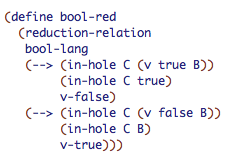Thanks to Erik Silkensen.

Page 225, in Racket version 6.4, reduction-relation's with clause changed, making this a syntax error. Use this definition instead (the only change is in the last line)

```(define iswim-standard
(reduction-relation
iswim
(v ((λ X M) V) (subst M X V) βv)
(v (o b ...) (δ (o b ...)) δ)
with
[(--> (in-hole E A) (in-hole E B)) (v A B)]))
```
Thanks to Mike MacHenry.

Page 230, exercise 13.2 should ask the reader to compare with the full grammar from exercise 12.2, not 12.1. Thanks to Mike MacHenry.

Page 243, the reference to part I(6.4) should be to partI(6.3).
Thanks to Leandro Facchinetti.

Page 253, the ISWIM program needs to read as follows:

```((Y (λ tri (λ x
((((iszero x)
(λ y 0))
(λ y (+ x (tri (- x 1)))))
0))))
3)
```

Thanks to Leandro Facchinetti.

Page 292 incorrectly claims that part I uses the notation M{x:=V} to specify the substitution of V for free x in M. Part I actually uses M[x<-V].
Relatedly, on page 294, it says that the type setting hooks add an extra space between M and [; this should be {.
Thanks to Leandro Facchinetti.